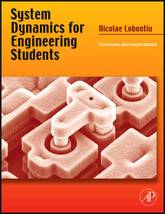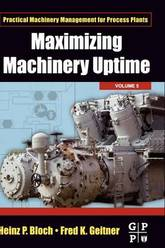Filter
(found 3 products)System Dynamics for Engineering Students: Concepts and Applications discusses the basic concepts of engineering system dynamics. Engineering system dynamics focus on deriving mathematical models based on simplified physical representations of actual systems, such as mechanical, electrical, fluid, or thermal, and on solving the mathematical models. The resulting solution is utilized ...
System Dynamics for Engineering Students
System Dynamics for Engineering Students: Concepts and Applications discusses the basic concepts of engineering system dynamics. Engineering system dynamics focus on deriving mathematical models based on simplified physical representations of actual systems, such as mechanical, electrical, fluid, or thermal, and on solving the mathematical models. The resulting solution is utilized in design or analysis before producing and testing the actual system. The book discusses the main aspects of a system dynamics course for engineering students; mechanical, electrical, and fluid and thermal system modeling; the Laplace transform technique; and the transfer function approach. It also covers the state space modeling and solution approach; modeling system dynamics in the frequency domain using the sinusoidal (harmonic) transfer function; and coupled-field dynamic systems. The book is designed to be a one-semester system-dynamics text for upper-level undergraduate students with an emphasis on mechanical, aerospace, or electrical engineering. It is also useful for understanding the design and development of micro- and macro-scale structures, electric and fluidic systems with an introduction to transduction, and numerous simulations using MATLAB and SIMULINK.
146.92 USD

## System Dynamics for Engineering Students

by Nicolae Lobontiu
HardbackThe authors use their decades of experience and draw upon real-world examples to demonstrate that the application of their techniques provides a basis for equipment management, uptime maximization, and reduced maintenance costs. The text explores reliability assessment techniques such as Failure Mode, Effect Analysis, and Fault Tree Analysis of commonly ...
Maximizing Machinery Uptime
The authors use their decades of experience and draw upon real-world examples to demonstrate that the application of their techniques provides a basis for equipment management, uptime maximization, and reduced maintenance costs. The text explores reliability assessment techniques such as Failure Mode, Effect Analysis, and Fault Tree Analysis of commonly encountered rotating machinery. These are all highly effective techniques that the engineer can apply to maximize uptime and thereby maximize production and profitability.